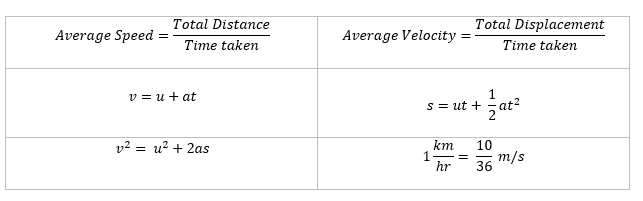# Class 9 Motion Notes (Physics) Chapter 8

Welcome to Class 9 Motion Notes for Chapter 8.The topics in this page are Motion along a straight line ,Speed & Velocity,Accelerations, Equations of uniformly accelerated Motion, Graphical representation of motion ,Equations of motion by graphical method and Uniform Circular Motion.This is according to CBSE and the NCERT textbook. If you like the study material, feel free to share the link as much as possible.

Table of Content

## 1. Introduction

In this page get class 9 motion notes in easy to understand language. This chapter is studied under class 9 physics. Concept of motion is foundation to all physics. you must have learned about motion and types of motion in physics from your previous classes. Here in class 9 physics chapter 8 of NCERT book we will study this topic in some more detail.

• In our daily life, we see lots of things moving around for example car passing through from one place to other, person riding on a bicycle and many more like this.
• In scientific terms an object is said to be in motion ,if it changes its position with the passage of time and if it does not change it position with the passage of time then it is said to be at rest.
• Both the motion and rest are relative terms for example mobile kept on the table is resting at its position but it is moving in the sense as earth is rotating on its axis. So for a person seeing mobile from earth it is at rest and for person on moon earth seems to change its position with time and so mobile is moving.
• Simplest case of motion is rectilinear motion which is the motion of the object in a straight line
• In our description of object, we will treat the object as a point object.
• Object under consideration can be treated as point object if the size of the object is much smaller than the distance traveled by it in reasonable time duration for example length of a motor car traveling a distance of 500km can be neglected w.r.t. distance traveled by it.

## 2. Motion along a straight line

• The simplest type of motion is the motion along a straight line.
• Two different quantities Distance and Displacement are used to describe the overall motion of an object and to locate its final position with reference to its initial position at a given time.
• Distance in physics, is the length of the path (the line or curve) described by an object moving through space. Distance is independent of direction. Thus, such physical quantities that do not require direction for their complete description are called scalars.
• When a body moves from one position to another the shortest distance between the initial and final position of the body along with its direction is known as displacement. Displacement has both direction and magnitude for its complete description and hence such physical quantities are called a vectors.
• The distance traveled by a moving body cannot be zero but the final displacement of a moving body can be zero.
• If a body covers equal distances in equal intervals of time then it is said to be having uniform motion.
• If a body covers unequal distances in equal intervals or equal distances in unequal intervals then body is said be having non-uniform motion.

### Concept Map of Motion in General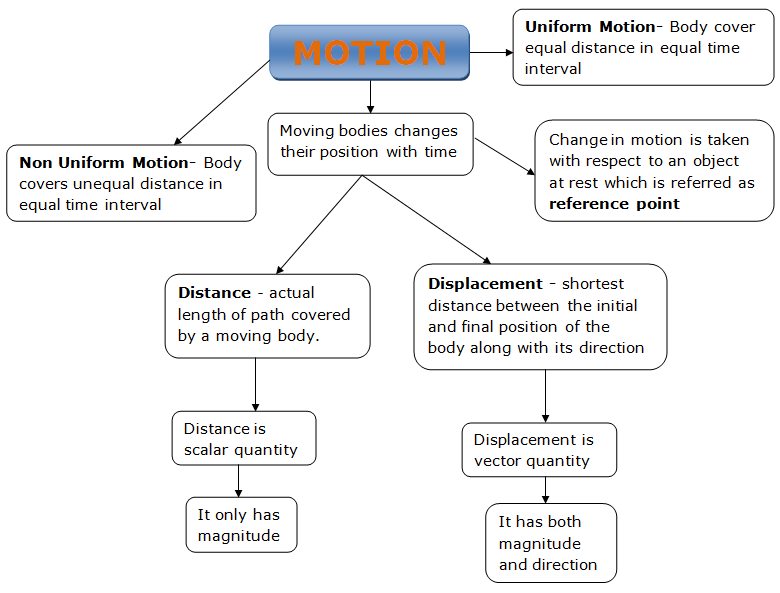Fill in the blanks
1. __________________ and ______ are used to describe the overall motion of an object and to locate its final position with reference to its initial position at a given time.
2. Physical quantities that do not require direction for their complete description are called ______
3. When a body moves from one position to another the shortest distance between the initial and final position of the body along with its direction is known as _______
4. Displacement has both direction and magnitude for its complete description and these physical quantities are called a _____
5. The distance travelled by a moving object cannot be zero but the final _______ of a moving object can be zero.
6. when the object covers unequal distances in equal intervals or equal distances in unequal intervals then motion is said be having ____ motion.
7. when body covers equal distances in equal intervals of time then it is said to be having _____________________.

## 3. Speed

• Speed is defined as the total distance travelled by the object in the time interval during which the motion takes place. SI unit of speed is meter per second. So,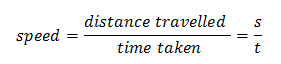where s is the distance traveled by the body and t is the time taken by the body to travel distance s.
• Speed of a body gives us the idea how slow or fast that body is moving.
• The ratio of total distance to total time taken by the body gives its average speed. The speed of a body at a given instant is its instantaneous speed.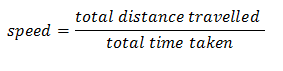• A body is said to have constant or uniform speed if it travels equal distance in equal intervals of time.

### Concept Map of Speed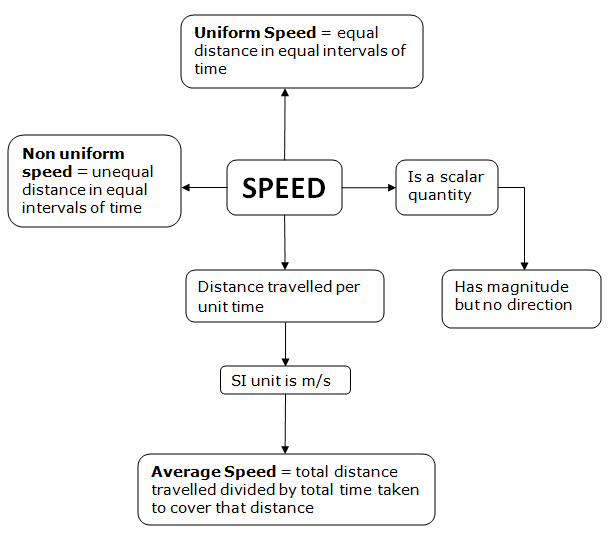## 4. Velocity

• The rate of change of displacement of a body with the passage of time is known as velocity of the body. Velocity of an object is measured in meter per second in SI units. So,• Velocity is nothing but the speed of an object moving in a definite direction.
• The velocity of an object can be uniform or variable. It can be changed by changing the object’s speed, direction of motion or both.
• So velocity of a body is a vector quantity involving both distance and displacement whereas speed of a body is a scalar quantity and it only has magnitude and does not have specific direction.
• Thus a body is said to be moving with uniform velocity if it covers equal distances in equal intervals of time in a specified direction.
• A body is said to be moving with non uniform velocity if it covers unequal distances in equal intervals of time and vice-versa in a specified direction or if it changes the direction of motion.
• The velocity of a body can be changed in two ways first by changing the speed of the body and second by changing the direction of motion of the body by keeping the speed constant. Also both speed and direction of the body can be varied in order to change the velocity of the body.
• When velocity of the object changes at a uniform rate, then average velocity is given by the arithmetic mean of initial velocity and final velocity for a given period of time. That is,
$\text{Average Velocity}= \frac {u + v}{2}$
Where u is the initial velocity of the object and v is the final velocity of the object.

### Concept Map of Velocity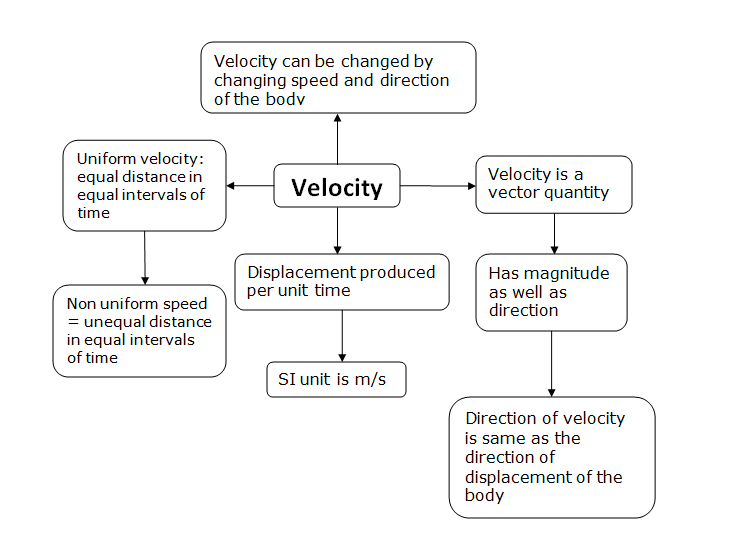## 5. acceleration

• Acceleration is a measure of the change in the velocity of an object per unit time and mathematically it is given as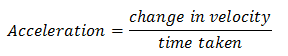• If the velocity of an object changes from an initial value u to the final value v in time t, the acceleration a is given by,
$a = \frac{{v - u}}{t}$
and this kind of motion is called accelerated motion.
• A body has uniform acceleration if it travels in a straight line and its velocity increases by equal amount in equal intervals of time for example freely falling bodies, motion of ball rolling down the inclined plane etc.
• A body has non uniform acceleration if its velocity increases or decreases by unequal amount in equal intervals of time.
• If acceleration is in the direction of the velocity then it is positive acceleration and if it is in the direction opposite to the direction of velocity then it is negative and the negative acceleration is termed retardation.
• SI unit of acceleration is ms-2.

### Concept Map of Acceleration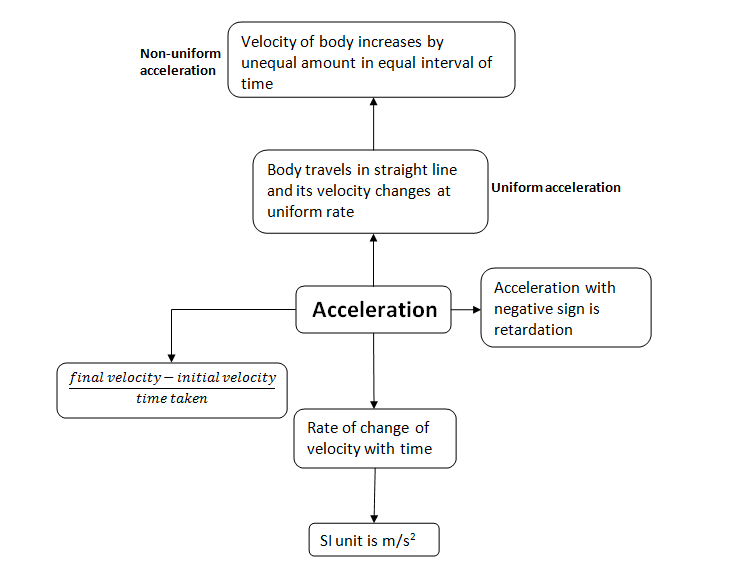### Speed, velocity and acceleration test questions

Fill in the Blank Questions
1. __________________ is defined as the total distance traveled by the body in the time interval during which the motion takes place.
2. __________________ unit of speed is m/s
3. The ratio of total distance to total __________________ taken by the body gives its average speed.
4. The rate of change of displacement of a object with the passage of time is known as __________________ of the object.
5. velocity of a body is a vector quantity involving both distance and __________________ whereas speed of a body is a scalar quantity and it only has magnitude and does not have specific direction.
6. A body is said to be moving with uniform velocity if it covers equal distances in equal intervals of time in a specified __________________.
7. A object is said to be moving with non uniform velocity if it covers unequal distances in equal intervals of time and vice-versa in a specified direction or if it
changes the direction of __________________.
8. If acceleration is in the __________________ of the velocity then it is positive acceleration and if it is in the __________________ opposite to the ___________
_______ of velocity then it is negative and the negative acceleration is termed retardation.

True and False Statement
1. Acceleration is a measure of the change in the speed of an object per unit time
2. A body has Non Uniform acceleration if its velocity increases or decreases by unequal amount in equal intervals of time.
3. When velocity of the object changes at a uniform rate, then average velocity is given by the arithmetic mean of initial velocity and final velocity for a given period of time.
4. The velocity of a body can be changed in two ways first by changing the speed of the object and second by changing the direction of motion of the body by keeping the speed constant.
5. The odometer in the car measures the velocity of the car
6. A object is said to have constant or uniform velocity if it travels equal distance in equal intervals of time.
7. A body has uniform acceleration if it travels in a straight line and its velocity increases by equal amount in equal intervals of time

## 6. Equations of uniformly accelerated motion

• There are three equations of bodies moving with uniform acceleration which we can use to solve problems of motion

### First Equation of motion

• The first equation of motion is $v = u + at$ , where v is the final velocity and u is the initial velocity of the body.
• First equation of motion gives velocity acquired by body at any time $t$.
• Now we know that acceleration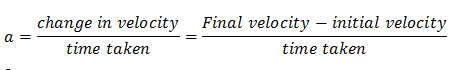so, $a = \frac{{v - u}}{t}$
and, $at = v - u$
rearranging above equation we get first equation of motion that is
$v = u + at$

### Second Equation of motion

• Second equation of motion is
$s = ut + \frac{1}{2}a{t^2}$
where $u$ is initial velocity, $a$ is uniform acceleration and $s$ is the distance traveled by body in time $t$.
• Second equation of motion gives distance traveled by a moving body in time $t$.
• To obtain second equation of motion consider a body with initial velocity $u$ moving with acceleration a for time $t$ its final velocity at this time be $v$. If body covered distance $s$ in this time $t$ , then average velocity of the body would be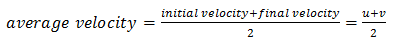Distance travelled by the body is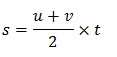From first equation of motion
$v = u + at$
So putting first equation of motion in above equation we get ,
$s = \frac{{u + u + at}}{2} \times t = \frac{{\left( {2u + at} \right)t}}{2} = \frac{{2ut + a{t^2}}}{2}$
Rearranging it we get
$s = ut + \frac{1}{2}a{t^2}$

### Third equation of motion

• Third equation of motion is
${v^2} = {u^2} + 2as$ where $u$ is initial velocity, $v$ is the final velocity, $a$ is uniform acceleration and $s$ is the distance travelled by the body.
• This equation gives the velocity acquired by the body in traveling a distance $s$.
• Third equation of motion can be obtained by eliminating time t between first and second equations of motion.
So, first and second equations of motion respectively are
$v = u + at$ and $s = ut + \frac{1}{2}a{t^2}$
Rearranging first equation of motion to find time t we get
$t = \frac{{v - u}}{a}$
Putting this value of t in second equation of motion we get
$s = \frac{{u\left( {v - u} \right)}}{a} + \frac{1}{2}a{\left( {\frac{{v - u}}{a}} \right)^2}$

$s = \frac{{uv - {u^2}}}{a} + \frac{{a\left( {{v^2} + {u^2} - 2uv} \right)}}{{2{a^2}}}$

$s = \frac{{2uv - 2{u^2} + {v^2} + {u^2} - 2uv}}{{2a}}$

Rearranging it we get
${v^2} = {u^2} + 2as$
• These three equations of motion are used to solve uniformly accelerated motion problems and following three important points should be remembered while solving problems
1. if a body starts moving from rest its initial velocity $u = 0$
2. if a body comes to rest i.e., it stops then its final velocity would be $v = 0$
3. If a body moves with uniform velocity then its acceleration would be zero.

### Test yourself for Equations of uniformly accelerated motion

1.The __________________ equation of motion is v=u+at , where v is the final velocity and u is the initial velocity of the body.
2.Second equation of motion is s=ut+1/2at2 where u is initial velocity, a is uniform acceleration and s is the ____________
______ travelled by body in time t.
3. Third equation of motion is v2=u2+2as where u is initial velocity, v is the final velocity, a is __________ and s is the distance traveled by the body.

## 7. Graphical representation of motion

• A graph is a pictorial representation of the relation between two sets of data of which one set is of dependent variables and the other set is of independent variables.
• To describe the motion of an object, we can use line graphs. In this case, line graphs show dependence of one physical quantity, such as distance or velocity, on another quantity, such as time.

### Distance Time Graphs

• The change in the position of an object with time can be represented on the distance-time graph.
• In this graph, time is taken along the x-axis and distance is taken along the y-axis.
• Distance time graphs of a moving body can be used to calculate the speed of the body as they specifically represent velocity.
• The distance time graph for a body moving at uniform speed is always a straight line as distance travelled by the body is directly proportional to time as shown below in the figure 1.
• The distance time graph for a body moving with non uniform speed is a curve and is shown below in the figure 2.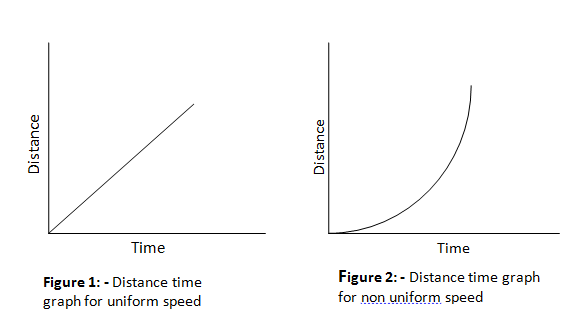• The distance time graph is parallel to time axis when the object is at rest and is shown below in figure 3.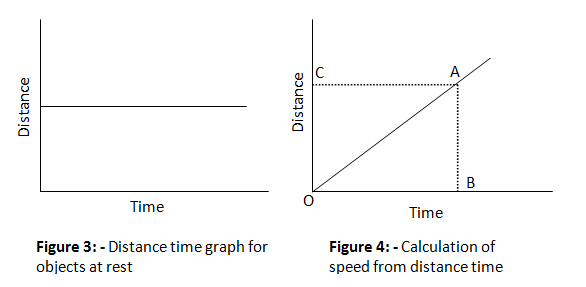• To calculate speed of the body from distance time graph say at point A first draw a perpendicular AB on time axis and a perpendicular $AC$ on distance axis so that $AB$ represents the distance travelled by the body in time interval OB and since we know that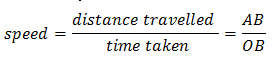### Velocity time graphs

• The variation in velocity with time for an object moving in a straight line can be represented by a velocity-time graph.
• In this graph, time is represented along the x-axis and the velocity is represented along the y-axis.
• The product of velocity and time give displacement of an object moving with uniform velocity. The area enclosed by velocity-time graph and the time axis will be equal to the magnitude of the displacement.
• If a body moves with a constant velocity then velocity time graph for this body would be straight line parallel to time axis as shown below in the figure 5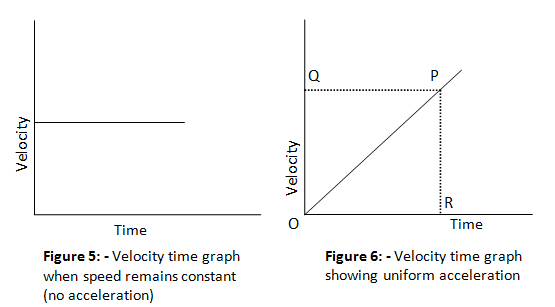• The velocity time graph of uniformly changing velocity is shown in figure 6 and is a straight line. We can find out the value of acceleration using the velocity time graph.
• For calculating acceleration at time corresponding to point R draw a perpendicular RP from point R as shown in figure 6 and we know that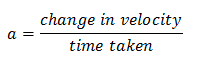Here change in velocity is represented by PR and time taken is equal to OR. So,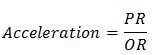which is equal to the slope of velocity time graph. So we conclude that slope of velocity time graph of moving body gives its acceleration.
• The distance traveled by moving body in a given time will be equal to area of triangle OPR as shown in figure 6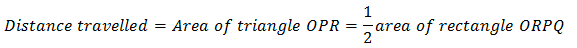so,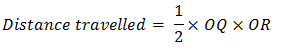• When the velocity of a body changes in an irregular manner then velocity time graph of the body is a curved line.

## 8. Equations of motion by graphical method

• We already know about equations of motion when an object moves along straight line with uniform acceleration. We already know how to derive them but these equations can also be derived by graphical method.

### a. Equation for velocity time relation

• Consider the velocity-time graph of an object that moves under uniform acceleration as shown below in the figure 7.
• From this graph, you can see that initial velocity of the object is $u$ (at point A) and then it increases to $v$ (at point B) in time $t$. The velocity changes at a uniform rate $a$.
• Again from figure it is clear that time $t$ is represented by OC , initial velocity $u$ by OA and final velocity of object after time $t$ by BC.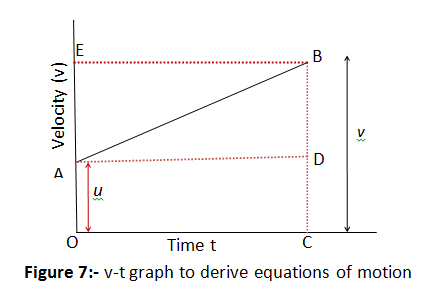• From graph as given in figure 7 it is clear that $BC = BD + DC = BD + OA$.
So we have
v=BD+u (1)
• We should now find out the value of BD. From the velocity-time graph (Fig. 7), the acceleration of the object is given by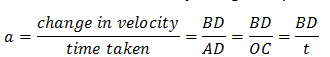which gives, $BD = at$
putting this value of BD in equation 1 we get
$v = u + at$
which is the equation for velocity time relation.

### b. Equation for position time relation

• Let us consider that the object has travelled a distance s in time t under uniform acceleration a. In Fig. 7, the distance travelled by the object is obtained by the area enclosed within OABC under the velocity-time graph AB.
• Thus, the distance s travelled by the object is given by
s = area OABC (which is a trapezium)
s= area of the rectangle OADC + area of the triangle ABD
So,
$s = OA \times OC + \frac{1}{2}\left) {AD \times BD} \right)$
Substituting $OA = u$, $OC = AD = t$ and $BD = at$, we get
$s = \left( {u \times t} \right) + \frac{1}{2} \times \left( {t \times at} \right)$
or,
$s = ut + \frac{1}{2}a{t^2}$
which is the equation of position time relation

### c. Equation for position velocity relation

• Again consider graph in figure 7. We know that distance travelled s by a body in time t is given by the area under line AB which is area of trapezium OABC. So we have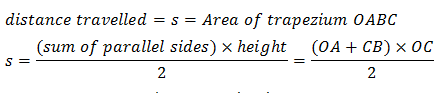Since $OA+CB=u+v$ and $OC=t$, we thus have
$s = \frac{{\left( {u + v} \right)t}}{2}$
From velocity time relation
$t = \frac{{v - u}}{a}$
putting this t in equation for s we get
$s = \frac{{\left( {u + v} \right)}}{2}\left( {\frac{{v - u}}{a}} \right)$
or we have
${v^2} = {u^2} + 2as$
which is equation for position velocity relation.

Fill in the blanks 1. The motion of an object moving at uniform acceleration can be described with the help of three equations, the equations are _____, ______ and __________
2. The change in the position of an object with time can be represented on the _______ graph.
3. The distance time graph for a body moving with non uniform __________________ is a curve
4. The motion of an object could be uniform or non-uniform depending on whether its velocity is constant or __________________.
5.The product of velocity and time give __________________ of an object moving with uniform velocity.
6. The area enclosed by velocity-time graph and the time axis will be equal to the magnitude of the ________
7. If a body moves with a constant velocity then velocity time graph for this body would be _____________ parallel to time axis
8. The acceleration of an object is the change in velocity per __________________time.
9. The value of _____________can be determined using the velocity time graph.________________ of moving body gives its acceleration.

## 9. Uniform circular motion

• When an object moves in a circular path at a constant speed then motion of the object is called uniform circular motion.
• In our everyday life ,we came across many examples of circular motion for example cars going round the circular track and many more .Also earth and other planets revolve around the sun in a roughly circular orbits
• If the speed of motion is constant for a particle moving in a circular motion still the particles accelerates because of constantly changing direction of the velocity.
• If an object moves in a circular path with uniform speed, its motion is called uniform circular motion
• Here in circular motion, we use angular velocity in place of velocity we used while studying linear motion.
• Force which is needed to make body travel in a circular path is called centripetal force.
• We know that the circumference of a circle of radius $r$ is given by $2\pi r$. If the body takes $t$ seconds to go once around the circular path of radius $r$, the velocity $v$ is given by
$v = \frac{{2\pi r}}{t}$
• One thing we must keep in mind is that uniform linear motion is not accelerated but uniform circular motion is accelerated motion.
• Examples of uniform circular motion are
(a) Motion of artificial satellites around the earth
(b) Moon, the natural satellite of earth, moves in uniform circular motion round the earth.
(c) Cyclist moving on a circular track with a constant speed exhibits uniform circular motion.

## Summary

Here is the Class 9 motion notes Summary
• The speed of an object is the distance covered per unit time, while velocity is the displacement per unit time.
• The acceleration of an object is the change in velocity per unit time
• When as object moves in a circular path with uniform speed, its motion is called uniform circular motion.
• General Formula used